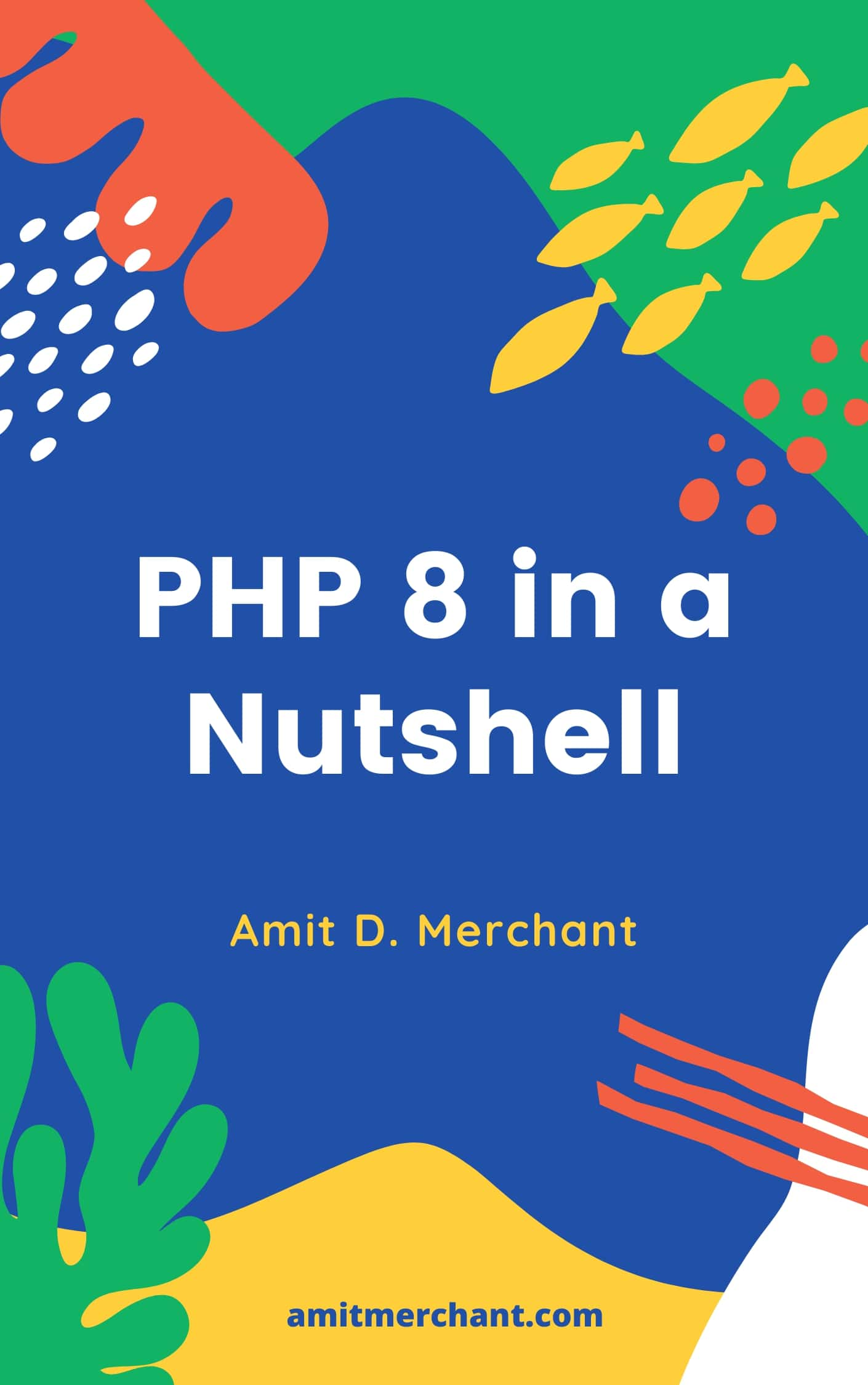# Amit MerchantVerified (\$10/year for the domain)

A blog on PHP, JavaScript, and more

# Safely convert any value to boolean in PHP

February 28, 2022 ·

Converting some values to boolean is a common task while building applications. Now, these values can be anything.

A pair of numbers. Let’s say 1/0. A pair of strings. Let’s say Yes/No or On/Off. Or even the checkbox’s checked/unchecked status. Scenarios are many.

## The problem

Now, if you want to convert any of these pairs to a boolean, you might end up using conditionals. Check the following for example.

``````\$is_public = \$_POST['is_public'] == 'Yes';
var_dump(\$is_public);
// true or false
``````

As you can tell, if we want to convert the Yes/No strings to a boolean value, we can write a conditional that checks the value against the string and it will return `true`/`false` accordingly.

This is fine. But there’s a handy way of doing this in PHP that I’m going to talk about in this article.

## The `filter_var` function

There’s a filter_var function in PHP that lets you filter a variable with a specified filter.

Here’s the definition of this function.

``````filter_var(
mixed \$value,
int \$filter = FILTER_DEFAULT,
array|int \$options = 0
): mixed
``````

As you can tell, the function accepts three parameters where the first parameter is the value that we want to be filtered. There other two are optional whereas the second one would be a predefined filter where we can provide one of the types of filter to filter the value against.

For our use case, we need to use the `FILTER_VALIDATE_BOOLEAN` filter along with the value.

So, if we want to rewrite the previous example using `filter_var`, here’s how we can do it.

``````// \$_POST['is_public'] could be Yes/No
\$is_public = filter_var(
\$_POST['is_public'],
FILTER_VALIDATE_BOOLEAN
);
var_dump(\$is_public);
// true for `Yes`
// false for `No`
``````

This works on a variety of different other values. Here are all the values that `filter_var` can effectively convert to their boolean counterpart.

value boolean
‘on’ bool(true)
‘On’ bool(true)
‘ON’ bool(true)
‘off’ bool(false)
‘Off’ bool(false)
‘OFF’ bool(false)
‘yes’ bool(true)
‘Yes’ bool(true)
‘YES’ bool(true)
‘no’ bool(false)
‘No’ bool(false)
‘NO’ bool(false)
0 bool(false)
1 bool(true)
‘0’ bool(false)
‘1’ bool(true)
‘true’ bool(true)
‘True’ bool(true)
‘TRUE’ bool(true)
‘false’ bool(false)
‘False’ bool(false)
‘FALSE’ bool(false)
true bool(true)
false bool(false)
‘foo’ NULL
‘bar’ NULL

Note here that `filter_var` will return `null` when it fails to convert the value to a boolean since we passed in `FILTER_NULL_ON_FAILURE` as the third parameter.

👋 Hi there! I'm Amit. I write articles about all things web development. If you like what I write and want me to continue doing the same, I would like you buy me some coffees. I'd highly appreciate that. Cheers!My Tiny YouTube Channel ⟶